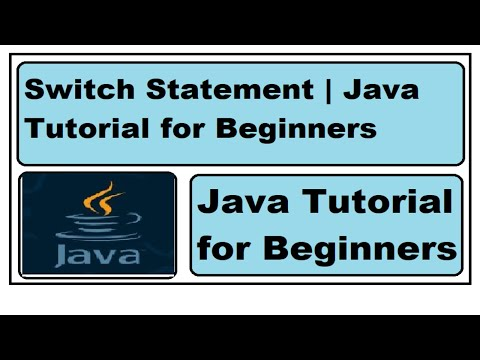## Monday, December 12, 2022

### Mathematical operations java | Arithmetic operators

In this tutorial we will learn how to use Arithmetic Operators, Mathematical operations in Java. we will create separate non static methods for each operation like addition, subtraction, multiplication, and division and we call those in main method. If you want to follow along by watching below is link for java basic calculator where we have used arithmetic operation by creating method. Step be Step tutorial is in English and Hindi Language.

Java Basic Calculator Video Tutorial in English

Java Basic Calculator Video Tutorial in Hindi

For more video click on this link @amazingcodingtechworld

First create class and checkmark on public static void, so we can have main method .

Create method, in this example we created method Add and we use void since we are not returning anything. After method name we use parenthesis and then curly brace where we use our logic inside. we created variable named a and datatype is integer and hold value of 5. After that we have created another variable b which datatype is integer and hold value of 10.  Since we are doing Addition we need result of both number that is why created another variable named result and we adding both number and we are printing it that is why we are using system.out.println as described in below image.

Now to call method in main class create instance of class . since we are using not static method we have to create instance of class. Syntax is name of class then variable then we use new word and class name then parenthesis. now if you use dot operator after that variable we will get all method which we can able to access. we want to use Add method so lets select it and check in output. we can observe we have result.

Now lets do Subtraction by creating non static method,  we have created method and created variable , a, b, and result . All have data type as integer and we are calling in main methods.

Now lets try out multiplication everything is same but in the result variable we have just changed operation.

Now we are doing last operation, division. so we are doing same thing created three variables and calling in the result we have changed operation we are doing division and we are calling method in main .

### java switch statement | Java Tutorial For Beginners

Switch statement is alternative and more cleaner way to write code if we have more than two options. we can use else if, this is better way...